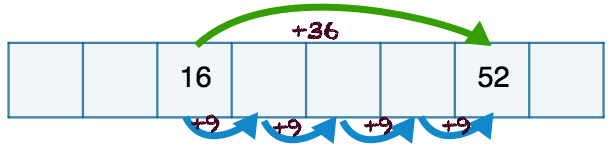# The general term, given two terms

In the figure below we are given two terms of an arithmetic sequence, namelyand.First, let’s find the common difference.

The difference between 52 and 16 is 36..

There are four ‘steps’ betweenand. So we divide the difference into four.The common difference between consecutive terms is.We can find the common difference using a calculation like this:Since we don’t know the first term in the sequence, we can either

• figure it out
• use a different technique.

Let’s use a different technique.

Since the common difference is 9, we know that the general term is. We don’t yet know the value of.

To calculate, let’s substitute one of our known terms:. Here, the term is 16 and it is in the third place, so.We can check this expression with the other known term. Let’s make sure that this expression gives us 52 for the seventh term.